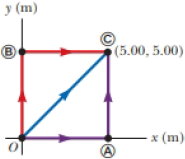# A force acting on a particle moving in the xy plane is given by F → = ( 2 y i ^ + x 2 j ^ ) , where F → is in newtons and x and y are in meters. The particle moves from the origin to a final position having coordinates x = 5.00 m and y = 5.00 m as shown in Figure P7.31. Calculate the work done by F → on the particle as it moves along (a) the purple path, (b) the red path, and (c) the blue path, (d) Is F → conservative or nonconservative? (e) Explain your answer to part (d). Figure P7.31### Physics for Scientists and Enginee...

10th Edition
Raymond A. Serway + 1 other
Publisher: Cengage Learning
ISBN: 9781337553278

#### Solutions

Chapter
Section### Physics for Scientists and Enginee...

10th Edition
Raymond A. Serway + 1 other
Publisher: Cengage Learning
ISBN: 9781337553278
Chapter 7, Problem 33P
Textbook Problem
3199 views

## A force acting on a particle moving in the xy plane is given by F → = ( 2 y i ^ + x 2 j ^ ) , where F → is in newtons and x and y are in meters. The particle moves from the origin to a final position having coordinates x = 5.00 m and y = 5.00 m as shown in Figure P7.31. Calculate the work done by F → on the particle as it moves along (a) the purple path, (b) the red path, and (c) the blue path, (d) Is F → conservative or nonconservative? (e) Explain your answer to part (d).Figure P7.31(a)

To determine

The amount of work done by the force along the purple path.

### Explanation of Solution

The coordinates of point (C) is (5.00m, 5.00m) and the force acting on the body is F=2yi^+x2j^.

Along path OA the work is done is only in x direction.

Write the expression for the work done along OA

WOA=05.00Fdxi^

Here, F is the force acting on the particle.

Substitute 2yi^+x2j^ for F in the above equation.

WOA=05.00(2yi^+x2j^)dxi^=05.00(2y)dx

The value y along path OA is 0.

Substitute 0 for y in the above equation.

WOA=05.00(2(0))dx=0J

Thus, work done along OA is 0J.

Write the expression for the work done along AC

WAC=05.00Fdyj^

Substitute 2yi^+x2j^ for F in the above equation

(b)

To determine

The amount of work done by the force along the red path.

(c)

To determine

The amount of work done by the force along the blue path.

(d)

To determine

Whether F is conservative.

(e)

To determine

The reason for the force F is not a conservative force.

### Still sussing out bartleby?

Check out a sample textbook solution.

See a sample solution

#### The Solution to Your Study Problems

Bartleby provides explanations to thousands of textbook problems written by our experts, many with advanced degrees!

Get Started

Find more solutions based on key concepts
To answer nutrition questions. a. watch for articles that include phrases such as "Now we know" or "The answer ...

Nutrition: Concepts and Controversies - Standalone book (MindTap Course List)

Give the molecular formula for each of the following structural formulas.

General Chemistry - Standalone book (MindTap Course List)

Velocity is a(n) ___ quantity. (2.2)

An Introduction to Physical Science

List the major types of lipoproteins.

Chemistry for Today: General, Organic, and Biochemistry

A star has a spectral class M. What type of star could this be?

Foundations of Astronomy (MindTap Course List)

Echo sounders bounce sound off the seabed to measure depth. How does that work?

Oceanography: An Invitation To Marine Science, Loose-leaf Versin

A barnacle is a shelled _______. a. arthropod b. gastropod c. cephalopod d. crustacean

Biology: The Unity and Diversity of Life (MindTap Course List)